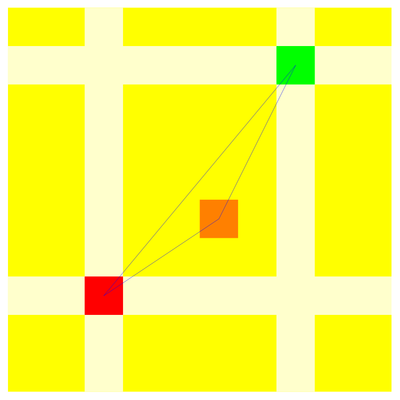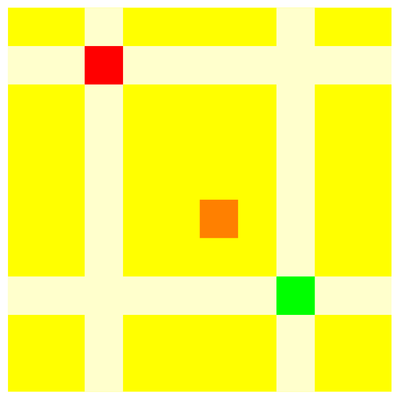M A T H 2 1 B
Mathematics Math21b Spring 2009
Linear Algebra and Differential Equations
Exhibit: Linear algebra in Comics: transpositions
Office: SciCtr 434

Some combinatorial insight is needed to see why switching two rows changes the number of upcrossings of a pattern by an odd number. Lets count the number of upcrossings before and after the switch.

Assume row a and c are switched. We look at one pattern and assume that(a,b) be an entry on row a and (c,d) is an entry on row b. The entry (a,b) changes the number of upcrossings to (c,d) by 1 (there is one upcrossing from (a,b) to (c,d) before which is absent after).

For each entry (x,y) inside the rectangle (a,c) x (b,d), the number of upcrossings from and to (x,y) changes by two. (there are two upcrossings to and from the orange squares before which are absent after).
For each entry outside the rectangle and different from (a,b),(c,d), the number of upcrossings does not change.